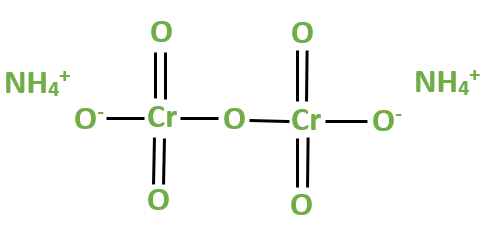Open in App
Not now

# Ammonium Dichromate Formula – Structure, Properties, Uses, Sample Questions

• Last Updated : 11 May, 2022

Ammonium Dichromate consists of elements Nitrogen, Hydrogen, Chromium, and Oxygen. Nitrogen is a nonmetal and one of the most common elements. Its atomic number is 7 and is preset in group-15 of the periodic table. It is the lightest member in its group. Nitrogen is represented with the symbol N. Hydrogen is odorless, tasteless, colorless, flammable, and the lightest gas. Its atomic number is 1 and is represented with the symbol H. Chromium is hard steely grey metal present in group-6 of the periodic table. Its atomic number is 24 and is represented by the symbol Cr. Chromium is corrosion resistance metal. Oxygen is a highly reactive nonmetal present in group-16 of the periodic table. Its atomic number is 8 and is represented by the symbol O.

### Ammonium Dichromate Formula

Ammonium Dichromate is an inorganic compound with the chemical formula (NH4)2Cr2O7. It is produced during crystallization as orange-red needles. It is a flammable compound. Ammonium Dichromate is a salt consisting of ammonium ions and dichromate ions. It is a toxic salt that sensitizes organic emulsion. It is more sensitive to light as it is readily ignited and burns to leave a sizable green residue. If ammonium dichromate is heated in a closed container then it may burst the container due to the decomposition. It is a reliable oxidizing agent.

Other names for Ammonium Dichromate are diammonium salt, Chromic acid, diammonium dichromate, Ammonium bichromate, and Ammonium pyrochromate. IUPAC name of Ammonium Dichromate is diammonium oxido-(oxido-dioxo-chromio) oxy-dioxo-chromium.

Structure of Ammonium DichromateAmmonium Dichromate Structure

Physical Properties of Ammonium Dichromate

• Ammonium Dichromate is odorless.
• It appears in the form of orange-red crystals.
• The melting point of Ammonium Dichromate is 180°C.
• Its autoignition temperature is 190°C.
• The molecular weight of Ammonium Dichromate is 252.07 g/mol.
• The density of Ammonium Dichromate is 2.12 g/cm3.

Chemical Properties of Ammonium Dichromate

• Ammonium Dichromate is soluble in alcohol but not soluble in acetone.
• On heating the Ammonium Dichromate solid it undergoes decomposition to give Chromium (III) oxide, nitrogen gas, and water vapor. The reaction of it is given below-

(NH4)2Cr2O7 → Cr2O3 + N2 + 4H2O

Uses of Ammonium Dichromate

• Ammonium Dichromate is used as a mordant for dyeing pigments, in the manufacturing of chrome alum, alizarin leather tanning, and oil purification.
• It is used as magnetic recording material.
• Ammonium Dichromate is an approved pesticide.
• Used to sensitize solutions in lithography.
• Acts as a catalyst in pyrotechnics.

### Sample Questions

Question 1: What happens if ammonium dichromate reacts with ethanol?

When few drops of ethanol is added to ammonium dichromate and ignite, a spark will emit and ash like powder will be formed. This reaction resembles the volcano eruption.

Question 2: Is Ammonium Dichromate ionic or covalent.

Ammonium Dichromate is considered as ionic compound. Because it features an ionic bond between Ammonium cation and dichromate anion. However the individual polyatomic cation and anion are held together by covalent bond.

Question 3: Find the molecular weight of Ammonium Dichromate.

Ammonium Dichromate has 2 nitrogen atoms, 8 hydrogen atoms, 2 chromium atoms and 7 oxygen atoms.

Atomic weight of each nitrogen = 14.0067

Atomic weight of each hydrogen = 1.00784

Atomic weight of each Chromium atom = 51.9961

Atomic weight of each oxygen atom = 15.999

Molar mass of (NH4)2Cr2O7 = (2 ×14.0067) + (8 × 1.00784) + (2 × 51.9961) + (7 × 15.999)

= 252.07

Question 4: What happens when Ammonium Dichromate is heated?

When heated, Ammonium Dichromate is decomposed into Chromium (III) Oxide, Nitrogen gas and water vapour.

(NH4)2Cr2O7 (Solid) → Cr2O3 (Solid) + N2 (Gas) + 4H2O (Gas)

Question 5: How Ammonium Dichromate reacts with reducing agents?